# Second Annual Meeting of Young Bulgarian Mathematicians

This meeting is the inaugural event of a series of annual meetings ICMS initiates. The series, which commemorates the brightest Bulgarian holiday the Day of Bulgarian Enlightenment and Culture and the Slavоnic Alphabet May 24, has as its main objective bringing together young Bulgarian mathematicians working all over the world.

We envision two main outcomes:

• enriching relations between young mathematicians working in Bulgaria and the Bulgarian mathematical diaspora;
• enhancing professional development of young Bulgarian mathematicians by presenting new opportunities using the national and European scientific programmes.

Following the conclusion of the scientific part of the event on May 19, we plan to organise a special round table to discuss these opportunities.

We are all elated by this exciting event and we are looking forward to seeing you there. This year the talks will happen via Zoom. We all hope to have an actual onsite live event starting next year.

## Invited speakers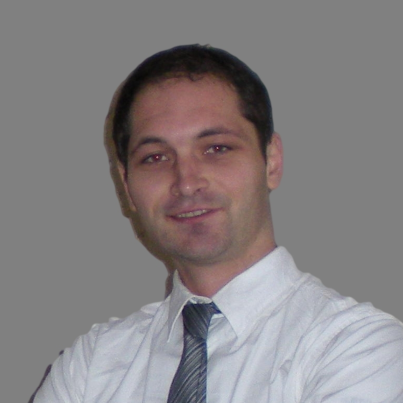## Alexander Petkov

### Sofia University “St. Kliment Ohridski”, Bulgaria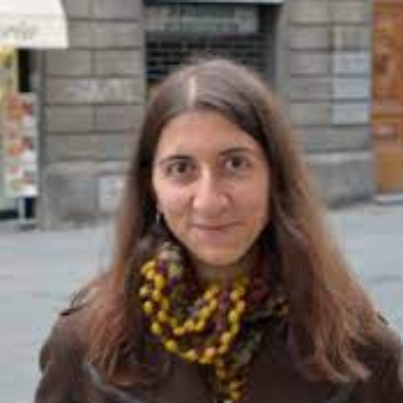## Alexandra Kjuchukova

### University of Notre Dame, USA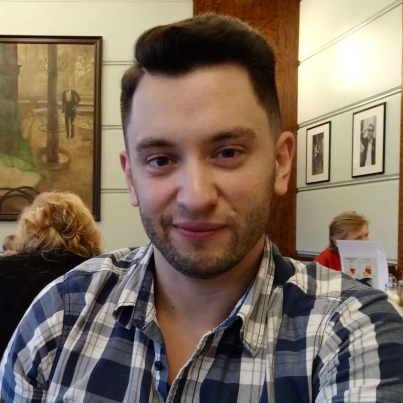## Antoni Rangachev

### University of Chicago, USA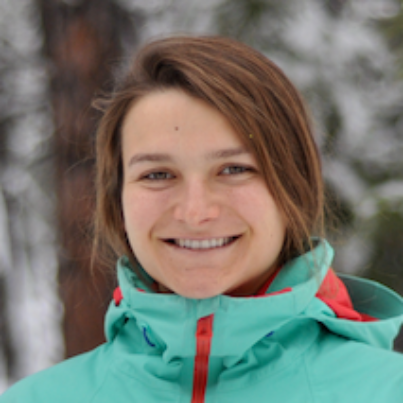## Elina Robeva

### University of British Columbia, Canada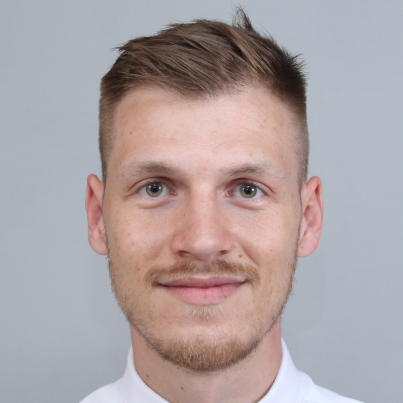## Hristo Sariev

### Institute of Mathematics and Informatics, Bulgarian Academy of Sciences, Bulgaria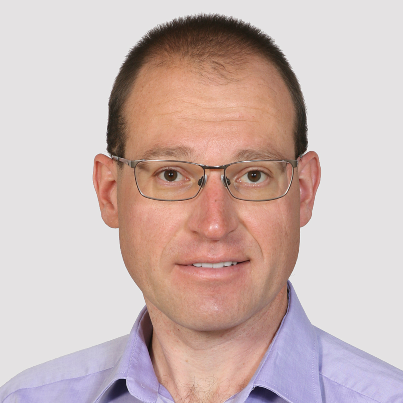## Kaloyan Slavov

### Swiss Federal Institute of Technology in Zürich, Switzerland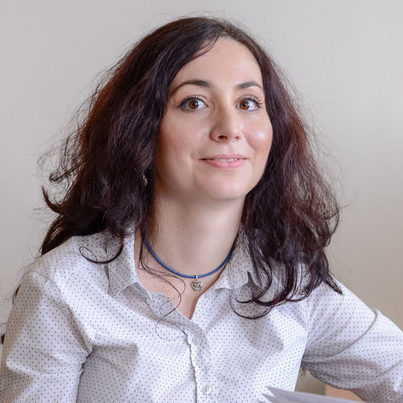## Mira Bivas

### Sofia University “St. Kliment Ohridski”, Bulgaria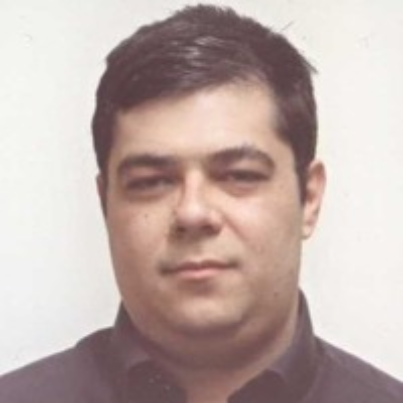## Afternoon session

Chair: Martin Kassabov, Cornell University, Ithaca, USA

#### 16:10-17:00 Kaloyan Slavov, Swiss Federal Institute of Technology in Zürich, Switzerland

Counting Irreducible Sparse Polynomials of a Given Degree over a Finite Field

Abstract: A classical result of Gauss states that among all monic polynomials of degree d over a finite field, approximately 1/d are irreducible. Extending previous results in the literature, we prove that under a mild assumption, the proportion of irreducible polynomials does not change even if only the last two coefficients are allowed to vary. Our approach is geometric. The talk will be nontechnical and accessible to a broad audience.

#### 17:10-18:00 Mira Bivas, Sofia University “St. Kliment Ohridski”, Bulgaria

Optimal Control for the Evolution of Deterministic Multi-agent Systems

Abstract: We investigate an optimal control problem with a large number of agents (possibly infinitely many). Although the dynamical system (a controlled ordinary differential equation) is of the same type for every agent, each agent may have a different control. So, the multi-agent dynamical system has two levels: a microscopic one, which concerns the control system of each agent, and a macroscopic level, which describes the evolution of the crowd of all agents. The
state variable of the macroscopic system is the set of positions of the agents. We define and study the evolution of such a global dynamical system whose solutions are called solution tubes. We also consider a minimization problem associated with the multi-agent system and we give a new characterization of the corresponding value function as the unique solution of a Hamilton- Jacobi-Bellman equation stated on the space of compact subsets of $R^d$.

The talk is based on joint work with Marc Quincampoix.

#### 18:10-19:00 Vesselin Dimitrov, University of Toronto, Canada

What is the smallest algebraic integer?

Abstract:  We survey Lehmer’s problem on the smallest Mahler measure of an integer non-cyclotomic polynomial, synthesizing an introduction to this subject along with the state-of-art results known today. Then we will present the solution of a closely related conjecture of Schinzel and Zassenhaus, and propose some answers towards the question of our title. Time permitting, we discuss also some related recent results on the geometry of integer polynomials.

## Round table

Moderators: Oleg Mushkarov and Antoni Rangachev

## Afternoon session

Chair: Kiril Datchev, Purdue University, USA

#### 16:00-16:50 Antoni Rangachev, University of Chicago, USA

Rigidity in Dimension 2

Abstract: I will discuss an approach to proving the conjecture that a normal rigid surface is smooth. The approach is based on the notion of deficient conormal singularities introduced by the speaker.

#### 17:00-17:50 Alexandra Kjuchukova, University of Notre Dame, USA

Coxeter quotients of knot groups

Abstract: A knot is a smooth embedding of a circle into the three-sphere. Knots have seduced the imagination since at least ancient Rome, and the classification of knots up to continuous deformations preoccupied even Gauss. Knots can be distinguished, and studied, using the fundamental groups of their complements in $S^3$. I will describe a method for computing the bridge number of a knot $K$ — a geometric invariant, which I will define — by using homomorphisms of $\pi_1(S^3\backslash K)$ onto Coxeter groups. This settles a conjecture by Cappell and Shaneson from the 70s for some infinite families.

Joint work with Sebastian Baader and Ryan Blair.

#### 18:00-18:50 Alexander Petkov, Sofia University “St. Kliment Ohridski”, Bulgaria

The Almost Schur Lemma in Quaternionic Contact Geometry

Abstract: In this talk we consider the quaternionic contact (qc) version of the almost Schur Lemma. Namely, we derive an integral inequality that informally states that on a compact qc manifold of dimension bigger than seven, satisfying some positivity condition, if the traceless qc Ricci tensor and some traces of the qc torsion tensor are close to zero, then the qc scalar curvature is close to a constant.

## Evening session

Chair: Greta Panova, University of Southern California, USA

#### 19:10-20:00 Elina Robeva, University of British Columbia, Canada

Orthogonal Tensor Decomposition

Abstract: Tensor decomposition has many applications. However, it is often a hard problem. In this talk we discuss a family of tensors, called orthogonally decomposable tensors, which retain many of the nice properties of matrices that general tensors don’t. A symmetric tensor is orthogonally decomposable if it can be written as a linear combination of tensor powers of n orthonormal vectors. We will see that the decomposition of such tensors can be found efficiently, their eigenvectors can be computed efficiently, and the set of orthogonally decomposable tensors of low rank is closed and can be described by a set of quadratic equations. Analogously, we study nonsymmetric orthogonally decomposable tensors, and show that the same results hold. Finally, we discuss a generalization to tight-frame decomposable tensors.

#### 20:10-21:00 Hristo Sariev, Institute of Mathematics and Informatics, Bulgarian Academy of Sciences, Bulgaria

Pólya Sequences with Dominant Colors

Abstract:

We introduce a class of  “Pólya Sequences with Dominant Colors”, which can be described as randomly reinforced urn processes with color-specific random weights and unbounded number of possible colors. Let ${D}$ be the set of colors for which the expected random reinforcement attains its maximum value; in this sense, we say that the colors in ${D}$ are dominant. Under fairly mild conditions, we show that the predictive probability of observing a dominant color, $P_n({D})$, converges a.s. to one. Moreover, there exists a random probability measure $\tilde{P}$ with $\tilde{P}({D})=1$ such that the predictive and the empirical distributions converge weakly a.s. to $\tilde{P}$. The latter implies, in particular, that the urn process is asymptotically exchangeable with limit directing random measure $\tilde{P}$. In the general case, for any $\delta$-neighborhood ${D}_\delta$ of ${D}$, the predictive probabilities $P_n({D}_\delta)$ and the empirical frequencies $\hat{P}_n({D}_\delta)$ converge a.s. to one. As a result, the distance between the observed color and ${D}$ converges in probability to zero. We refine the above results with rates of convergence and central limit theorems. We further hint examples of the potential use of our model in randomized clinical trials and species sampling.

• The conference will be held via Zoom. A direct link to the virtual hall, the conference protocol and some technical tips on Zoom are available below.

• All times are EET (UTC+2) – Sofia local time.